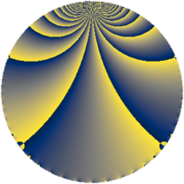Properties

 Label 144.1.gLevel $144$ Weight $1$ Character orbit 144.g Rep. character $\chi_{144}(127,\cdot)$ Character field $\Q$ Dimension $1$ Newform subspaces $1$ Sturm bound $24$ Trace bound $0$

Related objects

Defining parameters

 Level: $$N$$ $$=$$ $$144 = 2^{4} \cdot 3^{2}$$ Weight: $$k$$ $$=$$ $$1$$ Character orbit: $$[\chi]$$ $$=$$ 144.g (of order $$2$$ and degree $$1$$) Character conductor: $$\operatorname{cond}(\chi)$$ $$=$$ $$4$$ Character field: $$\Q$$ Newform subspaces: $$1$$ Sturm bound: $$24$$ Trace bound: $$0$$

Dimensions

The following table gives the dimensions of various subspaces of $$M_{1}(144, [\chi])$$.

Total New Old
Modular forms 13 1 12
Cusp forms 1 1 0
Eisenstein series 12 0 12

The following table gives the dimensions of subspaces with specified projective image type.

$$D_n$$ $$A_4$$ $$S_4$$ $$A_5$$
Dimension 1 0 0 0

Trace form

 $$q + O(q^{10})$$ $$q - 2 q^{13} - q^{25} + 2 q^{37} + q^{49} + 2 q^{61} - 2 q^{73} - 2 q^{97} + O(q^{100})$$

Decomposition of $$S_{1}^{\mathrm{new}}(144, [\chi])$$ into newform subspaces

Label Dim. $$A$$ Field Image CM RM Traces $q$-expansion
$a_{2}$ $a_{3}$ $a_{5}$ $a_{7}$
144.1.g.a $1$ $0.072$ $$\Q$$ $D_{2}$ $$\Q(\sqrt{-3})$$, $$\Q(\sqrt{-1})$$ $$\Q(\sqrt{3})$$ $$0$$ $$0$$ $$0$$ $$0$$ $$q-2q^{13}-q^{25}+2q^{37}+q^{49}+2q^{61}+\cdots$$